Hans Walser, 

Fibonacci triangle

# 1     The triangle

Figure 1 gives the Fibonacci triangle.Fig. 1: Fibonacci triangle

In Figure 2 we see an orthogonal arrangement.Fig. 2: Orthogonal arrangement

# 2     Notation

For the Fibonacci numbers we use the usual upper case notation: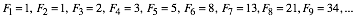(1)

For the Fibonacci triangle we will use the lower case notation of Figure 3.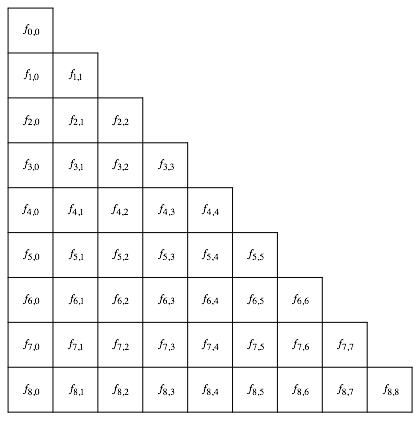Fig. 3: Notation

# 3     Features of the Fibonacci triangle

## 3.1    The Fibonacci numbers

The numbers in the first column of Figure 2 are the usual Fibonacci numbers:(2)

The Fibonacci numbers appear also under the roof: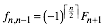(3)

## 3.2    Signs

The signs change every second step (Fig. 4).Fig. 4: Signs

## 3.3    Symmetry

The Fibonacci triangle is not symmetric. But leaving away the “roof” on the right and ignoring the signs there is an axial symmetry (Fig. 5).Fig. 5: Quasi symmetry

# 4     Powers of the Fibonacci numbers

## 4.1    Squares and cubes

Lets begin with a common picture (Fig. 6). It depicts the growth of the Fibonacci numbers. Beginning with a unit square we get the Fibonacci numbers for the side lengths of the square sequence. And of course we have the recursion: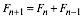(4)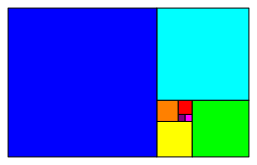Fig. 6: Fibonacci squares

For the areas of the squares we obtain the sequence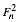:

1, 1, 4, 9, 25, 64, 169, 441, 1156, ...                                       (5)

Playing with these numbers we find a three digits recursion:(6)

Example:(7)

That means, that in Figure 6 the green and the cyan square have together half the area of the yellow plus the blue square. This can be seen by the dissection proof of Figure 7.Fig. 7: Dissection

Notice that the coefficients of (6) appear in the Fibonacci triangle: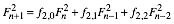(8)

For the cubesof the Fibonacci numbers we have:

1, 2, 8, 27, 125, 512, 2197, 9261, 39304, ...                                 (9)

Here we find a four digits recursion: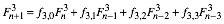(10)

Example: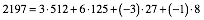(11)

## 4.2    General powers

For the kth power of the Fibonacci numbers there is a (k + 1) digits recursion:(12)

For the proof of (12) I used a computer algebra system.

# 5     The elements of the Fibonacci triangle

## 5.1    Columns

We have already found (2) the Fibonacci numbers in the first column of Figure 2.

In the next column of Figure 2 we have the products of two consecutive Fibonacci numbers:(13)

Example: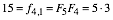(14)

Again in the next column we get products of three consecutive Fibonacci numbers, but with a negative sign an a coefficient of one half: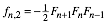(15)

Example:(16)

## 5.2    Overview

For the first five columns we get: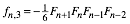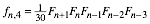The denominators in the coefficients are the products of consecutive Fibonacci numbers beginning with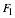(kind of “factorial”):(17)

## 5.3    General

Finally we get the general formula for the elements of the Fibonacci triangle: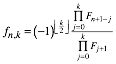(18)

# 6     Recursion in the Columns

The elements in the columns of the Fibonacci triangle fulfill a recursion like the recursion (12) for the powers of the Fibonacci numbers. Id est:(19)

Examples:

First example: n = 7, k = 1(20)

Figure 8 shows the involved elements. Red is the dot product of cyan and yellow.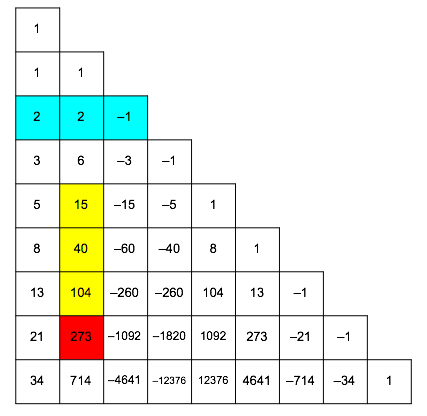Fig. 8: Dot product

Second example: n = 8, k = 2In Figure 9 the involved elements:Fig. 9: Involved elements